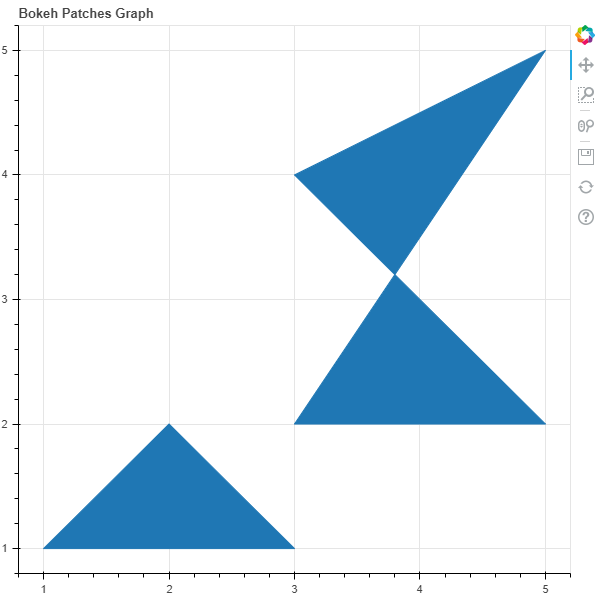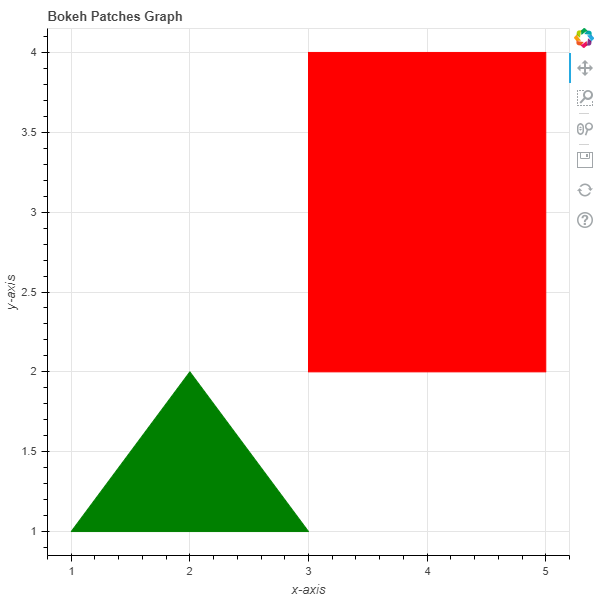# Python Bokeh – Plotting Multiple Patches on a Graph

Bokeh is a Python interactive data visualization. It renders its plots using HTML and JavaScript. It targets modern web browsers for presentation providing elegant, concise construction of novel graphics with high-performance interactivity.

Bokeh can be used to plot multiple patches on a graph. Plotting multiple patches on a graph can be done using the `patches()` method of the `plotting` module.

## plotting.figure.patches()

Syntax : patches(parameters)

Parameters :

• xs : x-coordinates of the patches
• ys : y-coordinates of the patches

Returns : an object of class `GlyphRenderer`

Example 1 : In this example we will be using the default values for plotting the graph.

 `# importing the modules  ` `from` `bokeh.plotting ``import` `figure, output_file, show  ` ` `  `# file to save the model  ` `output_file(``"gfg.html"``)  ` `      `  `# instantiating the figure object  ` `graph ``=` `figure(title ``=` `"Bokeh Patches Graph"``)  ` `    `  `# the points to be plotted ` `xs ``=` `[[``1``, ``2``, ``3``], [``3``, ``5``, ``3``, ``5``]] ` `ys ``=` `[[``1``, ``2``, ``1``], [``2``, ``2``, ``4``, ``5``]] ` `     `  `# plotting the graph  ` `graph.patches(xs, ys)  ` `      `  `# displaying the model  ` `show(graph) `

Output :Example 2 : In this example we will be plotting the patches with various other parameters

 `# importing the modules  ` `from` `bokeh.plotting ``import` `figure, output_file, show  ` ` `  `# file to save the model  ` `output_file(``"gfg.html"``)  ` `      `  `# instantiating the figure object  ` `graph ``=` `figure(title ``=` `"Bokeh Patches Graph"``)  ` ` `  `# name of the x-axis  ` `graph.xaxis.axis_label ``=` `"x-axis"` `      `  `# name of the y-axis  ` `graph.yaxis.axis_label ``=` `"y-axis"` ` `  `# points to be plotted ` `xs ``=` `[[``1``, ``2``, ``3``], [``3``, ``5``, ``5``, ``3``]] ` `ys ``=` `[[``1``, ``2``, ``1``], [``4``, ``4``, ``2``, ``2``]] ` ` `  `# color value of the patches ` `color ``=` `[``"green"``, ``"red"``] ` ` `  `# fill alpha value of the patches ` `fill_alpha ``=` `1` ` `  `# plotting the graph  ` `graph.patches(xs, ys, ` `              ``color ``=` `color, ` `              ``fill_alpha ``=` `fill_alpha)  ` `      `  `# displaying the model  ` `show(graph) `

Output :My Personal Notes arrow_drop_upCheck out this Author's contributed articles.

If you like GeeksforGeeks and would like to contribute, you can also write an article using contribute.geeksforgeeks.org or mail your article to contribute@geeksforgeeks.org. See your article appearing on the GeeksforGeeks main page and help other Geeks.

Please Improve this article if you find anything incorrect by clicking on the "Improve Article" button below.

Article Tags :

Be the First to upvote.

Please write to us at contribute@geeksforgeeks.org to report any issue with the above content.